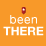# Geometric Network, Network Analyst, Utility Network Analyst, Network Datasets

3838
1
04-19-2012 11:30 AM
Labels (1)Occasional Contributor
What is the difference between them?

I want to be able to measure automatically, and quickly, the up/downstream distance between multiple sets of points (i.e. sample locations and dams/sewage) on a river network.   I cannot believe how difficult it is to figure out what the right tool is for the task, or if such a tool exists.

Here's what I think I've figured out:
1) A geometric network (which is managed using the Utility Network Analyst and/or Network Analyst???) can store a permanent flow direction like I would need for a river network.  But in order to calculate flow distances I have to manually click on the network, I can't do this for a feature class of a couple hundred points.
2) Using linear referencing I should be able to calculate these distances.
3) Linear referencing requires a Network dataset.
4) A network dataset does not contain built-in/permanent (flow)direction like a geometric network.

Am I anywhere near right?
Tags (2)
1 ReplybyEsri Regular Contributor
Some clarifications:
Geometric networks are for modeling utility networks such as electric, sewer, river, etc that have a concept of a flow direction. The utility analysis toolbar has tools that operate on geometric networks. You can create/analyse these networks without the need for any additional extension licenses.

Network datasets are for modeling transportation networks where you can have turn restrictions and cost attributes to help find paths. There is no concept of flow direction as people can travel where they want! The Network Analyst extension (licensed) is used to analyze network datasets.

In either kind of networks, linear referencing does not play a role. Linear Referencing is about having measure values at each vertex in addition to the X,Y coordinate. Their are tools to operate on such data. Primary users are the Dept of Transportation that collect data on top of linear referenced data.

Network Analyst solvers can output lines with measures. For example the shortest path based on length in miles from New York to Boston can have X,Y and M value where the M value is the Miles away from New York.

So if you have the concept of upstream/downstream, create a geometric network, set the flow direction:
http://help.arcgis.com/en/arcgisdesktop/10.0/help/index.html#/About_setting_flow_direction/002r00000...

and then use the utility network analysis toolbar to do a shortest path between points:
http://help.arcgis.com/en/arcgisdesktop/10.0/help/index.html#/Finding_the_shortest_path/002r00000037...

Jay Sandhu